# Ch-16 : Wave Shaping and Multivibrator Circuits¶

## Page No. 512 Example 16.1.¶

In :
print "Given      tr = 35 ns"
bw=0.35/(35*10**-9) # in Hz
x1=bw*10**-6  #in MHz
print "We know that,  tr = 0.35 / BW"
print "Therefore,    BW = 0.35 / tr =%0.2f MHz"%x1

Given      tr = 35 ns
We know that,  tr = 0.35 / BW
Therefore,    BW = 0.35 / tr =10.00 MHz


## Page No. 514 Example 16.2.¶

In :
print "Given      ton = 70 ns"
x1=C*10**12  # in pF
print "         C = ton / 0.1*Rs =%0.2f pF"%x1  # approximately 1200 pF
tre=2.3*(5.6*10**3)*(1200*10**-12) # in seconds
x2=tre*10**6  #in us
print "      tre = 2.3*RB*C =%0.2f u-seconds"%x2
f=1./(2*(15*10**-6))  #in Hz
x3=f*10**-3  #in kHz
print "      f = 1/2T = 1/2tre =%0.2f kHz"%x3

Given      ton = 70 ns
C = ton / 0.1*Rs =1166.67 pF
tre = 2.3*RB*C =15.46 u-seconds
f = 1/2T = 1/2tre =33.33 kHz


## Page No. 514 Example 16.3.¶

In :
from numpy import arange,sin,pi
%matplotlib inline
from matplotlib.pyplot import plot,subplot,title,xlabel,show,ylabel
amp = 15.
vi_t=3.# # transition voltage
t=arange(0,2*pi+0.1,0.1)
vi=[]
for x in t:
vi.append(amp*sin(x))
vo=[]
for x in vi:
vo.append(x+3)# # output voltage
print 'transition voltage: %0.2f'%vi_t
for i in range(0,len(t)):
if(vo[(i)]<=0):
vo[(i)]=0

subplot(2,1,1)
plot(t,vo,2,'011','',[0,0,7,18])
title('Ouptut voltage in sin wave')
xlabel('t')
ylabel('vo')
show()

t=arange(0,20+0.1,0.1)
vo=[]
for i in range(0,int(len(t)/2)):
vo.append(15+3)

for i in range(int(len(t)/2-1),len(t)-1):
vo.append(0)
subplot(3,1,2)

plot(t,vo,2,'011','',[0,-5,21,20])#
title('Ouptut voltage in square wave')
xlabel('t')
ylabel('vo')#
show()

transition voltage: 3.00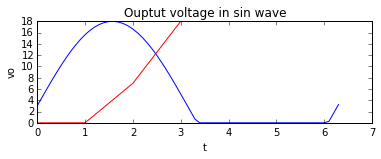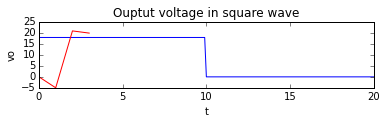## Page No. 515 Example 16.4.¶

In :
from numpy import arange
%matplotlib inline
from matplotlib.pyplot import plot,subplot,title,xlabel,show,ylabel

t= arange(0,20+0.1,0.1)
x=[]
for i in range(0,len(t)):
if(t[(i)]<=5):
x.append((15.0/5)*t[(i)])
elif(t[(i)]>=5 and t[(i)]<=15):
x.append(-3.2*t[(i)]+30)
elif(t[(i)]>=15 and t[(i)]<=20):
x.append((15./5)*t[(i)]-60)
y=[]
for i in range(0,len(t)):
if(x[(i)]>3):
y.append(x[(i)])
elif(x[i]<=3):
y.append(3)
plot(t,y,2,'011','',[0,0,20,16])#

title('output voltage')
xlabel('t')
ylabel('Vo')
show()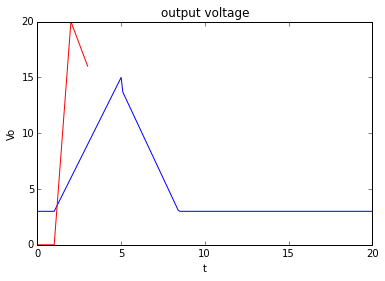## Page No. 516 Example 16.5.¶

In :
from numpy import arange
%matplotlib inline
from matplotlib.pyplot import plot,subplot,title,xlabel,show,ylabel,legend

#let input wave be V_in=V_p_in*sin(2*pi*f*t)
f=1.#    #Frequency is 1Hz
T=1./f#
V_p_in=10#    #Peak input voltage
V_th=0.7#    #knee voltage of diode
#let n be double the number of cycles of output shown in graph
for n in range(0,2):
t=arange(T*n/2.,T*(n+1)/2.+0.0005,0.0005)    #time for each half cycle
V_in=[]
for tt in t:
V_in.append(V_p_in*sin(2*pi*f*tt))
Vout=V_in#
if (n%2)==0: #positive half,D1 conducts till V_in=5V
a=(Vout<5)#
b=(Vout>5)#
y=[]
for vv in Vout:
y.append(a*vv+5*b)#    #output follows input till 5V then is constant at 5V
else:                    #negative half, D2 conducts till V_in=-3V
a=(Vout<-3)#
b=(Vout>-3)#
for vv in Vout:
y.append(-3*a+b*vv)  #output follows input till -3V then stays constant at -3V

plot(t,y[0:1001])
plot(t,V_in)
legend(['output','input'])
title('Positive and Negative diode limiter')
xlabel('t')
ylabel('Vo')
show()
print 'max output voltage is 5V'
print 'min output voltage is -3V'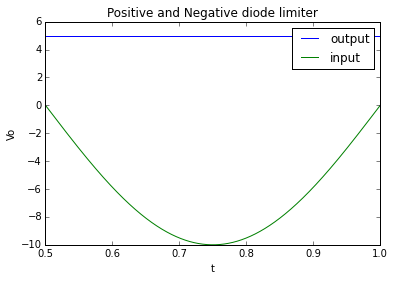max output voltage is 5V
min output voltage is -3V


## Page No. 518 Example 16.8.¶

In :
from numpy import arange,pi,sin
%matplotlib inline
from matplotlib.pyplot import plot,subplot,title,xlabel,show,ylabel
#Positive Clamping circuit
#let input voltage be V_in=V_p_in*sin(2*pi*f*t)
V_p_in=10.#
V_DC=(V_p_in)#    #DC level added to output
print 'V_DC = %0.2f V'%V_DC
for n in range(0,2):
t=arange(n/2,(n+1)/2+0.0005,0.0005)
V_in=[]
for tt in t:
V_in.append(V_p_in*sin(2*pi*tt))
Vout=[]
for vv in V_in:
Vout.append(V_DC+vv)
plot(t,Vout)

title('Positive clipper graph')
xlabel('t')
ylabel('Vo')
show()

V_DC = 10.00 V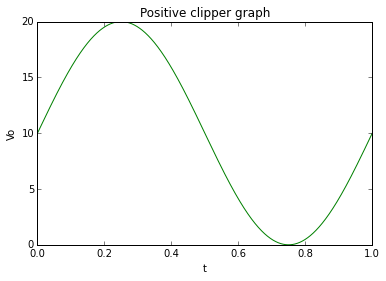## Page No. 519 Example 16.9.¶

In :
from numpy import arange,pi,sin
%matplotlib inline
from matplotlib.pyplot import plot,subplot,title,xlabel,show,ylabel
#Negative Clamping circuit
#let input voltage be V_in=V_p_in*sin(2*pi*f*t)
V_p_in=12#
V_DC=-(V_p_in)#    #DC level added to output
print 'V_DC = %0.2f V'%V_DC
#t=[]
for n in range(0,2):
t=(n/2.,(n+1)/2.+0.0005,0.0005)
V_in=[]
for tt in t:
V_in.append(V_p_in*sin(2*pi*tt))
Vout=[]
for vv in V_in:
Vout.append(V_DC+vv)
plot(t,Vout)

title('Negative clipper graph')
xlabel('t')
ylabel('Vo')
show()

V_DC = -12.00 V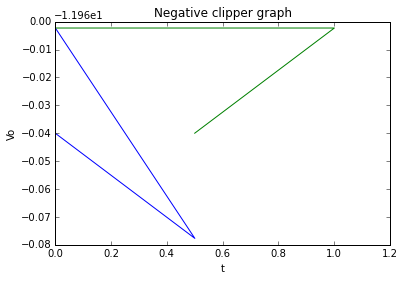## Page No. 520 Example 16.10.¶

In :
f=1./(1.386*(20*10**3)*(1000*10**-12))  #in Hz
x1=f*10**-3  # in kHz
print "The frequency of a symmetrical astable multivibrator is"
print "      f = 1/1.386RC =%0.2f kHz"%x1  # answer in textbook is wrong

The frequency of a symmetrical astable multivibrator is
f = 1/1.386RC =36.08 kHz


## Page No. 521 Example 16.11.¶

In :
print "The period of oscillation for an asymmetrical astable multivibrator is,"
t=0.693*(((2*10**3)*0.01*10**-6)+((10*10**3)*(0.05*10**-6))) # seconds
x1=t*10**6 # in us
print "    T = 0.693(R1C1+R2C2) = %0.2f us"%x1
f=1./(360.36*10**-6)  # in Hz
x2=f*10**-3 # in kHz
print "Therefore, the frequency of oscillation, f = 1/T =%0.2f kHz"%x2

The period of oscillation for an asymmetrical astable multivibrator is,
T = 0.693(R1C1+R2C2) = 360.36 us
Therefore, the frequency of oscillation, f = 1/T =2.78 kHz


## Page No. 522 Example 16.12.¶

In :
t=1./(100*10**3)  # in seconds
x1=t*10**6  # in us
print "The period of oscillation is,  T = 1/f = %0.2f us"%x1
print "        T1 = 2us  (given)"
t2=10-2 # in us
print "Hence,  T2 = T - T1 =%0.2f us"%t2
print "        T1 = 0.693*R1C1"
x1=c1*10**12  # in pF
print "Therefore,  C1 = T1 / 0.693R1 =%0.2f pF"%x1  #answer in textbook is wrong
x1=c2*10**12  # in pF
print "        T2 = 0.693*R2*C2"  #answer in textbook is wrong
print "Therefore,  C2 = T2 / 0.693R2 =%0.2f pF"%x1

The period of oscillation is,  T = 1/f = 10.00 us
T1 = 2us  (given)
Hence,  T2 = T - T1 =8.00 us
T1 = 0.693*R1C1
Therefore,  C1 = T1 / 0.693R1 =144.30 pF
T2 = 0.693*R2*C2
Therefore,  C2 = T2 / 0.693R2 =577.20 pF


## Page No. 523 Example 16.13.¶

In :
rc=(12-0.2)/(1*10**-3)  # in ohm
x1=rc*10**-3  # in k-ohm
print "  RC = 12-0.2/1*10**-3 = %0.2f kohm"%x1
r=100.*11.8*10**3  # in ohm
x1=r*10**-6  # in M-ohm
print "         R <= hfe*RC"
print "  R <=%0.2f Mohm"%x1
print "Hence, let us assume that R = R1 = R2 = 1 M-ohm"
print "    Toff = 0.693*R*C1"
x1=c1*10**12  # in pF
print "Therefore,  C1 =%0.2f pF"%x1
print "    Ton = 0.693*R*C2"
x1=c1*10**12  # in pF
print "Therefore,  C2 = %0.2f pF"%x1

  RC = 12-0.2/1*10**-3 = 11.80 kohm
R <= hfe*RC
R <=1.18 Mohm
Hence, let us assume that R = R1 = R2 = 1 M-ohm
Toff = 0.693*R*C1
Therefore,  C1 =28.86 pF
Ton = 0.693*R*C2
Therefore,  C2 = 14.43 pF


## Page No. 523 Example 16.14.¶

In :
print "At stable state, Q2 is ON and Q2 is OFF:"
rc2=(6.-0.3)/(6*10**-3) # in ohm
print "    RC2(ohm) = RC1(ohm) = VCC-VCE(sat) / IC(sat) =%0.2f ohm"%rc2
ib2=(6.*10**-3)/20 # in ampere
x1=ib2*10**3  # in mA
print "IB2(sat) = IC(sat) / hfe(min) =%0.2f mA"%x1
print "Also,    IB1(sat) = 0.3 mA"
r=(6-0.7)/(0.3*10**-3)  # in ohm
x1=r*10**-3  # in k-ohm
print "  R = VCC-VBE(sat) / IB2(sat) = %0.2f kohm"%x1
print "                                          [because, VBE(sat) = 0.7 V for Si transistor]"
print "At quasi-stable state, Q1 is ON and Q2 is OFF"
print "    T = 0.693*R*C"
c=(140.*10**-6)/(0.693*17.67*10**3)  # in F
x1=c*10**6  # in uF
print "Therefore,      C= T / 0.693*R =%0.2f uF"%x1
print "Assume,    IB1(sat) = IR2"
ir2=0.3+0.3  # in mA
print "Therefore,  IR1 = IB1(sat)+IR2 =%0.2f mA"%ir2
r1=((6-0.7)/(0.6*10**-3))-950  # in ohm
x1=r1*10**-3  # in k-ohm
print "    VCC = VBE(sat) + IR1(RC2+R1)"
print "Therefore,  R1 = (VCC-VBE(sat) / IR1) - RC2 =%0.2f kohm"%x1
r2=(0.7+1.5)/(0.3*10**-3)  # in ohm
x1=r2*10**-3  # in k-ohm
print "    R2 = VBE(sat)-(-VBB) / IR2 =%0.2f kohm"%x1
print "The speed up capacitor C1 is chosen such that R1C1 = 1 us and hence,"
c1=(1.0*10**-6)/(7.833*10**3)  # in F
x1=c1*10**12  # in pF
print "      C1 = %0.2f pF" %x1  # answer in textbook is wrong

At stable state, Q2 is ON and Q2 is OFF:
RC2(ohm) = RC1(ohm) = VCC-VCE(sat) / IC(sat) =950.00 ohm
IB2(sat) = IC(sat) / hfe(min) =0.30 mA
Also,    IB1(sat) = 0.3 mA
R = VCC-VBE(sat) / IB2(sat) = 17.67 kohm
[because, VBE(sat) = 0.7 V for Si transistor]
At quasi-stable state, Q1 is ON and Q2 is OFF
T = 0.693*R*C
Therefore,      C= T / 0.693*R =0.01 uF
Assume,    IB1(sat) = IR2
Therefore,  IR1 = IB1(sat)+IR2 =0.60 mA
VCC = VBE(sat) + IR1(RC2+R1)
Therefore,  R1 = (VCC-VBE(sat) / IR1) - RC2 =7.88 kohm
R2 = VBE(sat)-(-VBB) / IR2 =7.33 kohm
The speed up capacitor C1 is chosen such that R1C1 = 1 us and hence,
C1 = 127.67 pF


## Page No. 524 Example 16.15.¶

In :
vb1=(-12.*15*10**3)/(115.*10**3)  # in volts
print "      VB1 = -VBB*R2 / R2+R3 = %0.2f V"%vb1
ic2=((12-0.3)/(2.2*10**3))-((0.3+12)/(115*10**3)) # in A
x1=ic2*10**3  # in mA  (Since Q2 is ON VC2(sat) = 0.3 V)
print "          IC2 = [VCC-VC2(sat) / RC2] - [VC2(sat)-(-VBB) / R2+R3] =%0.2f mA"%x1             # answer in textbook is wrong
ib2=(5.35*10**-3)/20  # in A
x1=ib2*10**3  # in mA
print "      IB2 > IC2 / hfe(min) > %0.2f"%x1  # approximately 0.5 mA
i6=(0.7+12)/(100)  # in mA
print "Therefore,  I6 = %0.2f mA"%i6
i3=0.5+0.127  # in mA
print "            I3 =%0.2f mA"%i3
vc1=12-((0.627*10**-3)*(2.2*10**3))
print "            VC1 = %0.2f V"%vc1

      VB1 = -VBB*R2 / R2+R3 = -1.57 V
IC2 = [VCC-VC2(sat) / RC2] - [VC2(sat)-(-VBB) / R2+R3] =5.21 mA
IB2 > IC2 / hfe(min) > 0.27
Therefore,  I6 = 0.13 mA
I3 =0.63 mA
VC1 = 10.62 V


## Page No. 525 Example 16.16.¶

In :
ve=5-0.7  # in volts
print "Voltage across RE is VE = VB2 - VBE =%0.2f V"%ve
re=4.3/2 # in k-ohm
print "    RE = VE / IE =%0.2f kohm"%re
x=12-4.3-0.2  # in volts
print "     IC*RC2 = VCC - VE - VCE(sat) = %0.2f V"%x
rc2=7.5/(2)  # in k-ohm
print "     RC2 =%0.2f kohm"%rc2
i2=0.1*2  # in mA
print "     I2 = 0.1*IC2 =%0.2f mA"%i2
r2=5/0.2  # in k-ohm
print "     R2 = VB2 / I2 =%0.2f kohm"%r2
ib2=(210**-3)/100  # in A
x1=ib2*10**6  # in uA
print "     IB2 = IC2 / hfe(min) = %0.2f uA"%x1
x=7/(0.22)  # in k-ohm
print "RC1 + R1 =%0.2f"%x
i1=3./25 # in mA
print "  I1 = VB2 / R2 =%0.2f mA"%i1
ic1=(3-0.7)/2.15  # in mA
print "  IC1 = IE = VB1-VBE / RE =%0.2f mA"%ic1
rc1=(12-((0.12*10**-3)*(56.8*10**3)))/(1.07*10**-3)  # in  ohm
x1=rc1*10**-3  # in k-ohm
print "Therefore,  RC1 =%0.2f kohm"%x1
r1=31.8-4.84
print "  R1 = %0.2f kohm"%r1
rb=(100*2.15)/10
print "  RB < hfe*RE"
print "  RB = hfe*RE / 10 =%0.2f kohm"%rb

Voltage across RE is VE = VB2 - VBE =4.30 V
RE = VE / IE =2.15 kohm
IC*RC2 = VCC - VE - VCE(sat) = 7.50 V
RC2 =3.75 kohm
I2 = 0.1*IC2 =0.20 mA
R2 = VB2 / I2 =25.00 kohm
IB2 = IC2 / hfe(min) = 0.00 uA
RC1 + R1 =31.82
I1 = VB2 / R2 =0.12 mA
IC1 = IE = VB1-VBE / RE =1.07 mA
Therefore,  RC1 =4.84 kohm
R1 = 26.96 kohm
RB < hfe*RE
RB = hfe*RE / 10 =21.50 kohm